• ## 计算理论

千次阅读 2018-05-11 18:27:38
一，计算模型：1. 有穷(状态)自动机确定型 有穷自动机定义：由5元组组成，字母集，状态集，转移函数，初始状态，接受状态如果A是机器M接受的全部字符串集，则称A是机器M的语言，记做L(M)=A。也叫M识别A。A={w|M接受w...
一，计算模型：1. 有穷(状态)自动机确定型 有穷自动机定义：由5元组组成，字母集，状态集，转移函数，初始状态，接受状态如果A是机器M接受的全部字符串集，则称A是机器M的语言，记做L(M)=A。也叫M识别A。A={w|M接受w}如果一个语言被一台有穷自动机识别，则称它为正则语言。注意：一般情况描述：机器接受字符串，机器识别语言，M接受w, M识别A给定某个语言，如何设计一台确定型有穷自动机？设计 确定型 有穷自动机是一个创作过程，没有公式可言。先要根据语言特征来确定有哪些状态，然后再确定转移函数。正则语言的运算：并，连接和星号。A并B运算=两个集合求并A连接B运算=A和B笛卡尔积的集合A的星号运算=A的0次方并上A的1次方并上A的2次方...。0次方是空串，1次方是A本身，2次方是A连接A...定理：两个正则语言求并得到的新语言也是正则语言，连接运算和星号运算也是同理。有穷自动机分为确定型DFA和非确定型NFA，每一台NFA都有一台等价的DFA，构造NFA比构造DFA代价小。集合A的幂集是指A的所有子集的集合。一个正则语言，有且仅有一台（非）确定型有穷自动机识别。2.正则表达式是使用正则运算符（并，连接，星号）把元素（字母表集合或字符串）组合起来的表达式R。{0,1}*001{0,1}*该表达式能够表达含有001字符串的语言。L(R)=A语言。如何判断一个语言是不是正则的，可以使用泵引理进行证明（？）是正则语言，则一定可以设计出相应的正则表达式或有穷自动机，相反非正则语言就是正则表达式和有穷自动机的局限性。有穷自动机和正则表达式一般应用于文本处理，编译程序的词法分析器，硬件设计（如：超市自动门） 3.上下文无关的文法定义：由四元组组成（1.变元集（2.终结符集（3.替换规则或产生式：变元 (大写字母表示) ->字符串（由终结符 (小写字母表示) 和变元组成）（4.起始变元根据上下文无关的文法由起始变元推出的所有由终结符组成的字符串的集合叫做上下文元关的语言。有穷自动机能识别的语言，  上下文无关的文法也一定能识别。 反之则不然。根据调用不同顺序的替换规则，可能产生不同的语法分析树，但最终派生出相同的字符串，说明该文法是有歧义的，解决办法是规则推导顺序，有最左推导和最右推导。根据乔姆斯基范式生成的上下文无关的文法是简单简洁的。设计上下文无关的文法也是需要创造力，没有公式可言。上下文无关的文法一般用于程序设计语言的规范说明和编译程序的语言法分析器4.下推自动机下推自动机(识别器，主要看某语言的字符串能否被识别)的能力与上下文无关的文法（生成器，主要看文法能生成哪些字符串）等价二，可计算性理论图灵机三，复杂性理论
展开全文• ## 计算理论导引

千次阅读 2019-04-30 22:05:31
计算理论导引 计算机的基本能力和限制是什么？ 计算理论 自动机 可计算性 复杂性 计算复杂性理论 复杂性理论的核心问题：是什么使得某些问题很难计算，又使得另一些问题容易计算？ 复杂性理论的一个重要成果：...
计算理论导引

计算机的基本能力和限制是什么？
计算理论

自动机
可计算性
复杂性

计算复杂性理论

复杂性理论的核心问题：是什么使得某些问题很难计算，又使得另一些问题容易计算？
复杂性理论的一个重要成果：发现一个按照计算难度给问题分类的完美体系。
面对难题：1. 搞清问题困难的根源，改变问题条件变成简单问题，2.近似解，3.问题在最坏情况下困难，随机计算。
密码学需要复杂性
目标是把问题分成容易的和困难的

可计算性理论

哥德尔，图灵，丘奇
确定一个数学命题的真假
计算机理论模型
目标是把问题分成可解的和不可解的

自动机理论

自动机理论论述计算的数学模型的定义和性质
有穷自动机
上下文无关文法
正则表达式

数学基础

集合
函数
谓词（性质）：是值域为真假10的函数
关系：一种谓词，为多元函数（定义域为多元笛卡尔集），如二元关系，大于，小于，等于，等价关系（传递，自反，对称）
图集合G(V,E)：路径，子图，树，最小生成树，无向图，联通，有向图，强连通
字符串：字母表为有穷集（如（0,1），（a-z）26字母），字符串为字母表集族，如0101010，方法：空串，长度，子串，反转，连接，字典序（长短），字符序（a,b,）,
语言:语言是字符串的集合
实数集：字母表：0-9，字符串为自然数集合或者整数，有理数集合，集族在构成实数集
布尔逻辑：与或非，与（合取），或（析取），异或（逻辑加），同或。德摩根定律
定义：定义是数学的灵魂，（欧式几何的定义），定理和证明是数学的精髓，对象+概念=数学命题
定理：是被证明为真的数学命题，（引理[lemma]:辅助定理）
证明;逻辑论证，证明命题为真，
如何证明（如何解题）：归纳（递归），演绎，反证法，启发式，构造性证明（存在性证明）

第一部分：自动机与语言
第二章 正则语言
计算理论的第一个问题是：

什么是计算机？

采用计算模型作为理论计算机，如图灵机。$\lambda$ 演算，有穷状态机（有穷自动机：存储有限，状态有限），马尔科夫链

有穷自动机

形式定义：计算的形式定义：​     正则语言：能被有穷自动机识别的语言，（语言是字符串的集合）
设计有穷自动机：状态信息，数字逻辑电路的设计，策略就是“读者就是自动机”
正则运算：并，连接，*号，正则语言类在并，连接，运算下封闭
非确定性：
确定性有穷自动机（DFA）
非确定性有穷自动机（NFA），DFA为NFA子集NFA的形式定义：NFA的计算：
NFA等价于DFA

正则表达式：用正则运算符构造描述语言的表达式，如UNIX 的AWK, GREP,PERL语言等
形式定义：正则表达式与有穷自动机等价

非正则语言

上下文无关语言CFL

上下文无关文法CFG：替换规则（产生式），变元，终结符，派生，语法分析树形式定义：编译程序的语法分析

设计上下文无关文法

歧义性

乔姆斯基范式下推自动机

与上下文无关文法等价，为非确定型有穷自动机加栈组成，可识别某些非正则语言非上下文无关语言

第二部分 可计算性理论
第四章 丘奇-图灵论题

图灵机递归可枚举语言：图灵可识别
递归语言：图灵可判定
变化中的不变性：稳健性，鲁棒性，
图灵机的变形

非确定性图灵机

枚举器

算法的定义

希尔伯特第十问题：不定方程的整数根的算法求解

丘奇使用$\lambda$演算的记号系统来定义算法

丘奇-图灵论题：算法的非形式概念和精确概念的联系，用图灵机定义了算法的概念
图灵机刻画了所有的算法

第五章 可判定性

研究算法求解问题的能力

可判定语言停机问题
第六章 可归约性

第七章：可计算性理论的高级专题

递归定理

制造能生产自己的机器是可能的
自引用
信息的定义：算法与信息是计算机科学中基本的概念，用可计算性定义信息，极小长度的描述，描述复杂性

逻辑理论

图灵可归约性

描述复杂性

第三部分 复杂性理论

如果需要复杂的时间或者空间，即使可判定的理论可解问题也是不可解的

研究计算问题所需要的时间，空间或者其他资源的理论

度量复杂性

大欧记号，算法时间复杂度

P类问题

多项式时间NP类

多项式可验证性
coNP

NP完全性第九章 空间复杂性
第十章  难解性

近似算法
概率算法


展开全文• ## 计算理论总结

千次阅读 2018-01-22 16:55:35
计算理论复习 正则语言与有穷自动机 可数无穷 正则表达式（注意+不是正则，*是正则； L∅=∅L=∅L \emptyset = \emptyset L = \emptyset） Σ⋃{(,),∅,⋃,∗}\Sigma \bigcup \lbrace(,),\emptyset, \bigcup,* \...
计算理论复习

正则语言与有穷自动机

可数无穷
正则表达式（注意+不是正则，*是正则； L∅=∅L=∅$L \emptyset = \emptyset L = \emptyset$）
Σ⋃{(,),∅,⋃,∗}$\Sigma \bigcup \lbrace(,),\emptyset, \bigcup,* \rbrace$
DFA
M$M$ = (K$K$, Σ$\Sigma$, δ$\delta$, s$s$, F$F$) where
K$K$ is a finite set of states,
Σ$\Sigma$ is an alphabet,
s∈K$s \in K$ is the initial state,
F⊆K$F\subseteq K$ is the set of final states,
δ$\delta$ is the transition function form K×Σ$K \times \Sigma$ to K$K$
NFA
M$M$ = (K$K$, Σ$\Sigma$, Δ$\Delta$, s$s$, F$F$) where
K$K$ is a finite set of states,
Σ$\Sigma$ is an alphabet,
s∈K$s \in K$ is the initial state,
F⊆K$F\subseteq K$ is the set of final states,
Δ$\Delta$ is the transition relation form K×(Σ⋃{e})×K$K \times (\Sigma\bigcup\lbrace e\rbrace) \times K$ to K$K$
for each NFA, there is an equivalent DFA
(将NFA的状态的集合变为DFA中的点)
closure 和 pumping theory
The class of languages accepted vy finite automata is closed under:
union
concatentenation
Kleene star
complementation
intersection
A language is regular if and only if it is accepted by finite automaton
正则表达式和DFA， NFA的相互转化按照步骤生成
Pumping Theory： Let L$L$ be a regular language. There is an integer n≥1$n \geq 1$ such that any string w∈L$w \in L$ with |w|≥n$|w|\geq n$ can be rewritten as w=xyz$w = xyz$ such that y≠e,|xy|≤n$y \neq e, |xy|\leq n$, and xyiz∈L$xy^iz \in L$ for each i≥0$i \geq 0$

Give DFA or NFA write Regular Expression
Give regular expression, write DFA or NFA
Show a given language is (construct) or is not regular   (pumping)

context-free and pushdown自动机

context-free grammar
G$G$ = (V$V$, Σ$\Sigma$, R$R$, S$S$) where
V$V$ is an alphabet
Σ$\Sigma$ is the set of terminals, is a subset of V$V$
R$R$ is the set of rules (V−Σ)×V∗$(V - \Sigma) \times V^*$
S$S$ is the start Symbol, is an element of V−Σ$V - \Sigma$
all regular languages are context-free
parse tree, leftmost derivation, rightmost derivation
Grammars with strings that have two or more distinct parse trees are called ambiguous
PDA

M$M$ = (K$K$, Σ$\Sigma$, Γ$\Gamma$, Δ$\Delta$, s$s$, F$F$) where
K$K$ is a finite set of states
Σ$\Sigma$ is an alphabet (the input symbols)
Γ$\Gamma$ is an alphabet (the stack symbols)
s∈K$s \in K$ is initial state
F⊆K$F \subseteq K$ is the set of final states
Δ$\Delta$ is the transition relation (K×(Σ⋃{e})×Γ∗)×(K×Γ∗)$(K \times (\Sigma \bigcup \lbrace e \rbrace) \times \Gamma ^*) \times (K \times \Gamma ^*)$
The class of languages accepted by PDA is exactly the class of context-free languages
The transitions from CFL to PDA:

((p,e,e),(q,S))$((p, e, e), (q, S))$
((q,e,A),(q,x))$((q, e, A), (q, x))$ for each rule A→x$A\rightarrow x$ in R$R$
((q,a,a),(q,e))$((q, a, a), (q, e))$ for each a∈Σ.$a \in \Sigma .$
clousure, pumping theory
CFL are closed under union, concatenation, Kleene star, CFL在补和交上不封闭
CFL与正则的交集为CFL
pumping theory: Let G$G$ = (V$V$, Σ$\Sigma$, R$R$, S$S$). Then any string w∈L(G)$w \in L(G)$ of length greater than ϕ(G)|V−Σ|$\phi(G)^{|V-\Sigma|}$ can be written as w=uvxyz$w = uvxyz$ in such a way that either v$v$ or y$y$ is nonempty and uvnxynz$uv^nxy^nz$ is in L(G)$L(G)$ for every n≥0$n \geq 0$

Given context-free language, write context-free grammar and PDA
Give context-free grammar, write PDA
Show a given language is or is not Context-free

Turing Machine and Recursive Enumerable Language

Turing machine

M$M$ = (K$K$, Σ$\Sigma$, δ$\delta$, s$s$, H$H$) where
K$K$ is a finite set of states
Σ$\Sigma$ is an alphabet, containing the blank symbol ⨆$\bigsqcup$  and the left end symbol ⊳$\vartriangleright$, but not containing the symbol →$\to$ and ←$\leftarrow$
s∈K$s \in K$ is the initial state
H⊆K$H \subseteq K$ is the set of halting states
δ$\delta$, the transition function (K−H)×Σ$(K - H) \times \Sigma$ to K×(Σ⋃{→,←})$K \times (\Sigma \bigcup \lbrace \to, \leftarrow \rbrace)$
一些图灵机的符号表达(左右平移机(S←,S→$S_\leftarrow, S_\rightarrow$), 复制机)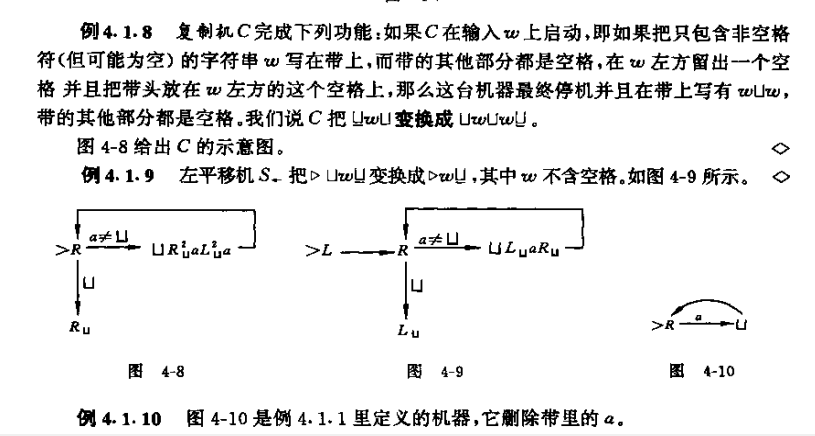M$M$ decide L$L$: if w∈L$w \in L$ then M$M$ accepts w$w$, and if w∉L$w \notin L$ then M$M$ rejects w$w$
Call a language recursice if there is a TM decides it.
A function f$f$ is called recursive if there is a TM computes f$f$
M$M$ semidecides L$L$: w∈L$w \in L$ iif M$M$ halts on input w$w$
Call a language recursicely enumerable iif there is a TM semidecides it.
if a language is recursive, then it is recursively enumerable
the complement of recursive language is also recursive
多带和复合
Corollary: Any function that is computed or language that is decided or semidecided by a k-tape TM is also computed, decided or semidecided by a standard TM.
Grammar
G$G$ = (V$V$, Σ$\Sigma$, R$R$, S$S$) where
V$V$ is an alphabet
Σ$\Sigma$ is the set of terminals, is a subset of V$V$
R$R$ is the set of rules (V∗(V−Σ)V∗)×V∗$(V^*(V - \Sigma)V^*) \times V^*$
S$S$ is the start Symbol, is an element of V−Σ$V - \Sigma$
a language is generated by a grammar iif it is recursively enumerable
递归可枚举只在补和差下不封闭
G$G$ computes f$f$, if: SwS⇒∗Gv$SwS \Rightarrow ^*_Gv$ iif v=f(w)$v = f(w)$.
A function f$f$ is called grammatically computable iif there is a grammar G$G$ computes it.
A fucntion f$f$ is recursive iif it is grammatically computable
the transition form TM to Grammar? (P230)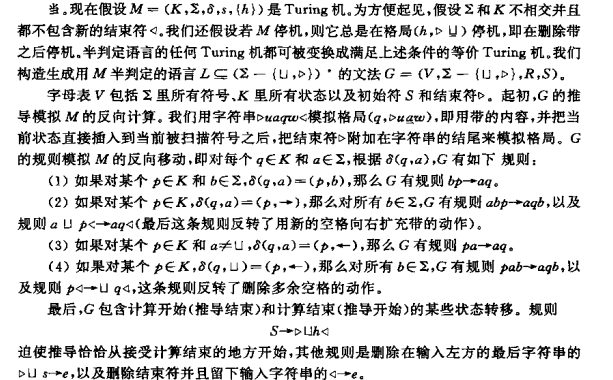Numerical Function
Basic Function:
zero, zerok(n1,n2,...,nk)=0$zero_k(n_1, n_2, ... ,n_k) = 0$
identity, idk,j(n1,n2,...,nk)=nj$id_{k,j}(n_1, n_2, ... ,n_k) = n_j$
successor, succ(n)=n+1$succ(n) = n + 1$
The primitive recursive functions are all basic functions, and all functions that can be obtained by them by any number of successive application of composition and recursive definition
other primitive resursive funstion:
plus(m,n)=m+n$plus(m, n) = m + n$ use m+n$m + n$
mult(m,n)=m∗n$mult(m, n) = m * n$ use m∗n$m * n$
exp(m,n)=mn$exp(m, n) = m^n$ use m↑n$m \uparrow n$
all constant functions of the form f(n1,...,nk)=17$f(n_1,..., n_k) = 17$
sgn(n)$sgn(n)$ which is zero if n=0$n = 0$
m∼n=max{m−n,0}$m \sim n = max\lbrace m-n,0\rbrace$ (defined use a predecessor function pred(0)=0,pred(n+1)=n$pred(0) = 0, pred(n+1) = n$)
primitive recursive predicate (primitive functions that only takes values 0 and 1):
greater−than(m,n)$greater-than(m,n)$, greater−than−or−equal(m,n)$greater-than-or-equal(m,n)$, equal(m,n)$equal(m,n)$
iszero(n)$iszero(n)$, isone(n)$isone(n)$, positive(n)$positive(n)$
Those function’s negation, disjunction and conjunction are also primitive recursive predicates
Funstion defined by cases:(can be written as)
f(n)=p(n)∗g(n)+(1∼p(n))∗h(n)$f(n) = p(n)*g(n) + (1 \sim p(n))*h(n)$
rem(m,n)$rem(m,n)$ and div(m,n)$div(m,n)$
not all computable functions are primitive recursive
denote minimalization of g$g$ by μ$\mu$ m[g(n1,n2,...,nk,m)=1]$m[g(n_1,n_2, ... ,n_k, m) = 1]$ The obvious method:
m:=0$m := 0$;
while g(n1,n2,...nk,m)≠1$g(n_1, n_2, ... n_k, m) \neq 1$ do m:=m+1$m:= m+1$
output m$m$
But it is not a algorithm becaues it may fail to terminate
call a function g$g$ minimalizable if the above method always terminates.
Call a function μ$\mu$-recursive if it can be obtained form basic functions by operations of composition, recursive definition, and minimalization of minimalizable funtions.
log(m,n)=μ p[greater−than−or−equal((m+2)↑p,n+1)]$log(m,n) = \mu \space p[greater-than-or-equal((m+2)\uparrow p, n+1)]$
A function is μ$\mu$-recursive iif it is recursive(that is, computable by a TM)

Design Turing machine to compute a function or decide (semidecide) a language
判断原始递归函数

Undecidablity

Church Turing Thesis
在所有输入上停机 ⇔$\Leftrightarrow$ 算法
Chomasky hierachy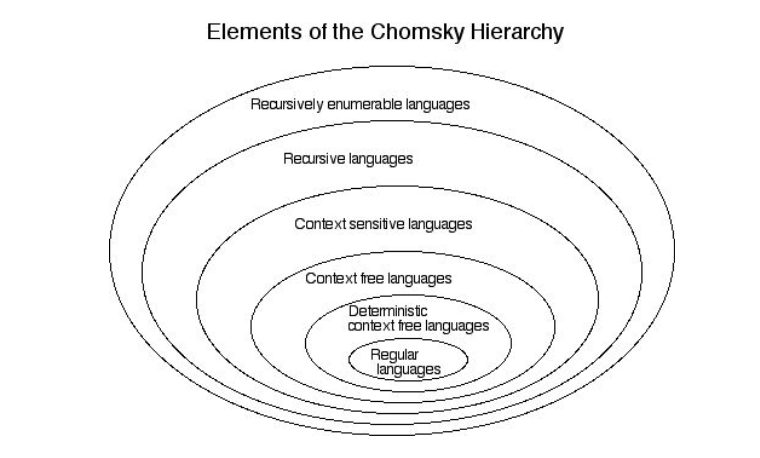Universal Turing Machine
状态{q}{0,1}∗$\lbrace q \rbrace \lbrace 0,1 \rbrace^*$
符号{a}{0,1}∗$\lbrace a \rbrace \lbrace 0,1 \rbrace^*$
特殊符号:⨆, ⊳, ←, →$\bigsqcup ,\space \vartriangleright ,\space \leftarrow ,\space \rightarrow$ 四个字典序下最小的符号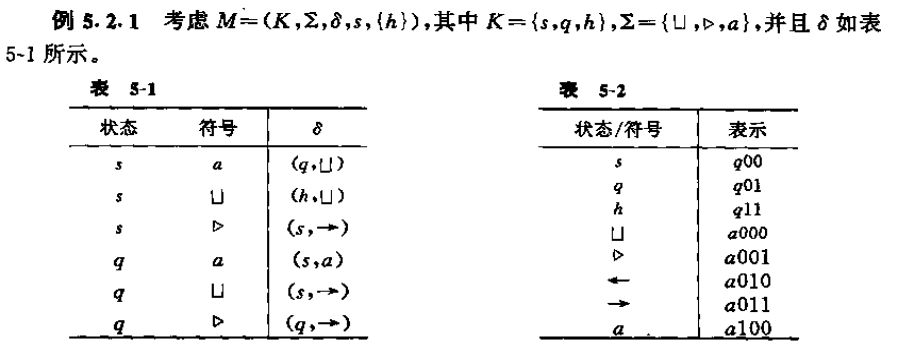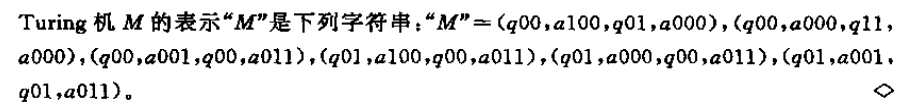通用图灵机Halting problem
H={"M","w":$H = \lbrace "M","w":$ TM M$M$ halts on input string w}$w \rbrace$ is recursively enumerable It is precisely the language semidecided by universal TM U.
The language H$H$ is not Recursive; therefore, the class of recursive languages is a strict subset of the class of recursively enumerable languages.
The class of recursively enumerable languages is not closed under complement
Some Undecidable problem and Reduction
若有X≤Y$X \leq Y$（X规约到Y）， X 不可判定，则 Y 不可判定；Y 可判定， 则 X 可判定。
Properites of recursive languages
A lanuage is recursive iif both it and its complement are recursively enumerable
Turing-enumerable and lexicographioally Turing enumerable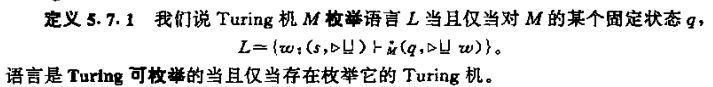A language is Turing-enumerable iif there is a TM enumerates it.
A language is recursively enumerable iif it is turing-enumerable.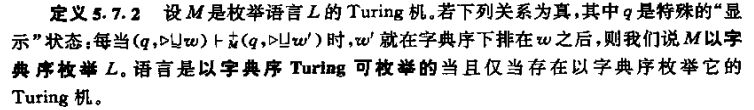A language is recursive iif it is lexicographically Turing-enumerable
Rice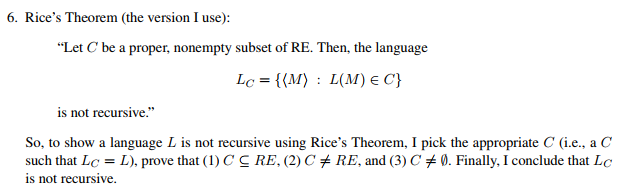Show a given language be recursive enumerable
show a given language be not recursive last one

来自总结的一些例子：

a∗b∗c∗−{anbncn,n>=0}$a^*b^*c^* -\lbrace a^nb^nc^n, n >= 0 \rbrace$ is context free but not regular
L=L1L2$L = L_1L_2$, L$L$是context free, 则L1$L_1$ 一定是context free  (x)
Regular - context free不一定是CFL (如a∗b∗c∗−anbn$a^*b^*c^* - a^nb^n$包含anbncn$a^nb^nc^n$)
2-way PDA(i.e. PDA whose input head can move both left and right are more powerful than 1-way PDA
Given a PDA M1$M_1$ and an FA M2$M_2$, the problem L(M1)⊆L(M2)$L(M_1) \subseteq L(M_2)$ is deciable
非正则语言的kleen star也可能是正则的。
正则语言的子集也可能非正则。
递归与μ$\mu$递归等价
PDA中，若每一个格局至多有一个格局接在它后面，则为确定型的。确定型CFL在补下封闭
A countable union of regular languages is necessarily regular (x) 可数包含可数无穷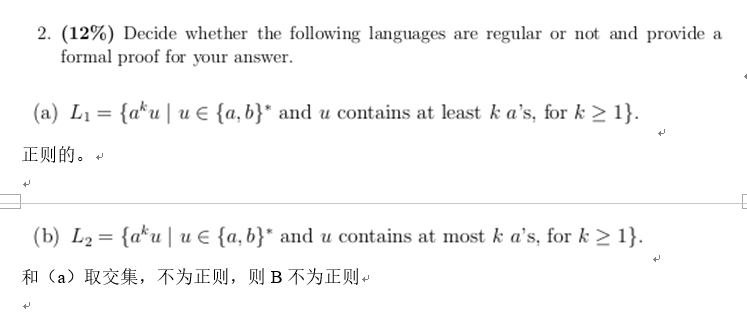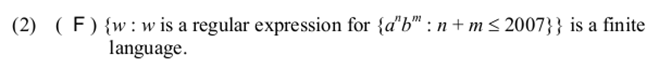有时构造context-free不太好想时，通过构造PDA证之。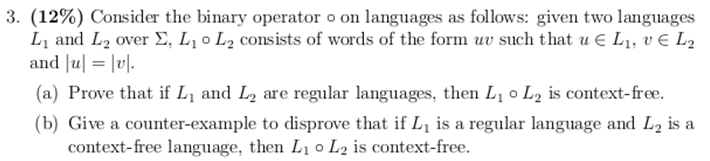It is decidable whether or not a given string belongs to a context-free language.
It is decidable whether or not a context-free language is empty.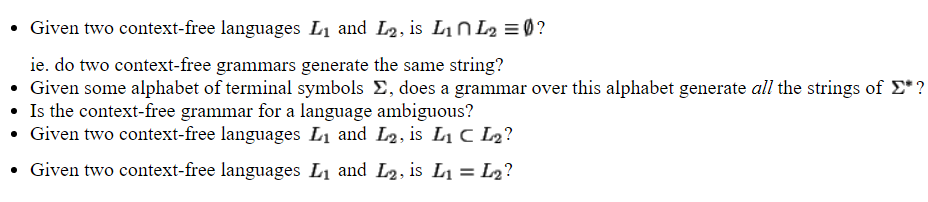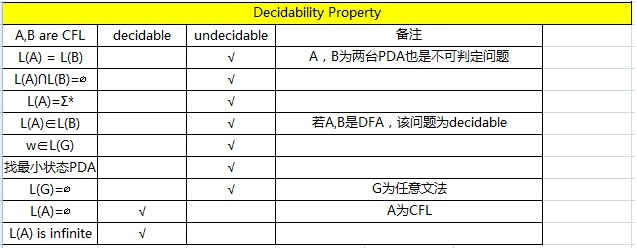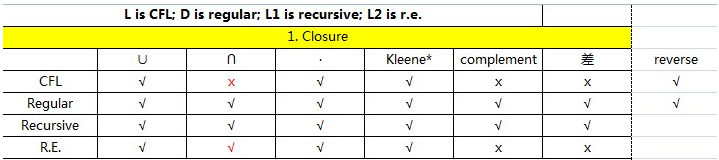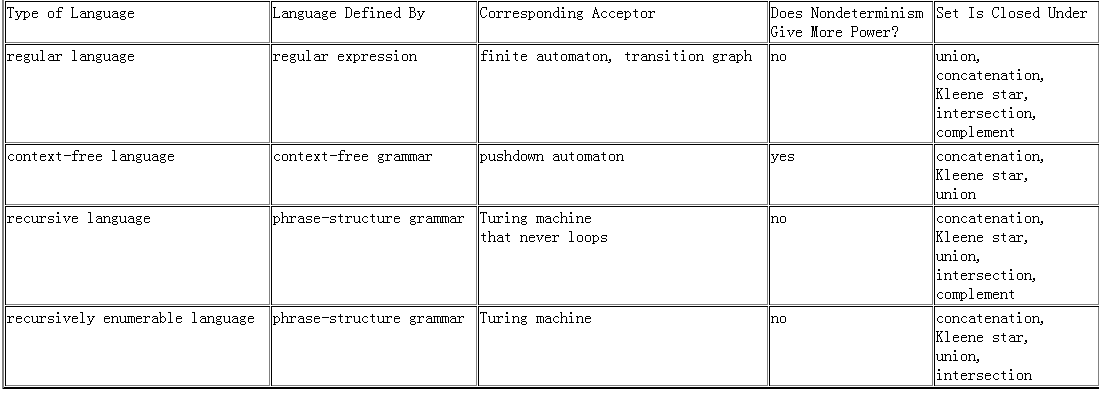pumping theory不能反过来证明是否正则或CFL
If L1$L_1$ is regular, L2$L_2$ is not regular, then L1∘L2$L_1 \circ L_2$ must be non-regular (X)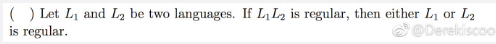判断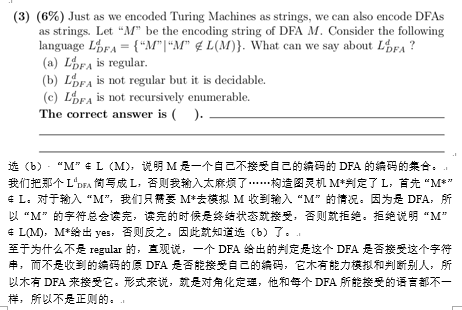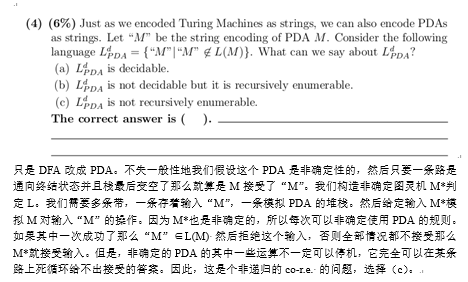对于非确定图灵机的n步，确定图灵机要用n的指数步来模拟
判断下面问题是否可判定
参考链接

两个基本问题(前者为递归可枚举但不递归，后者不是递归可枚举)一个图灵机至少有481个状态 （YES）
给定图灵机在空串上走了481步还没有停机 （YES）
给定图灵机,判断它是否在一些输入上经过481步还没有停机 （YES）
给定图灵机，判断它是否在所有输入上经过481步还没有停机 （YES）
给定图灵机是否接受空串 （NO）
给定TM M, 是否存在在M上停机的串？ （NO）
给定TM M，M是否在所有串上停机？ （NO）
给定TM M，is L(M) finite？ （NO）(通过取非？)
给定TM M， 带上是否出现过a(a∈Σ$\in\Sigma$)？ （NO）
给定M1,M2$M_1, M_2$，他们是否在同一个字符串上停机？ （NO）
给定M， 只要M接受w, M接受wR$w^R$  （NO）
{M||L(M)|>2}.$\lbrace M| |L(M)|>2 \rbrace.$ 递归可枚举但不递归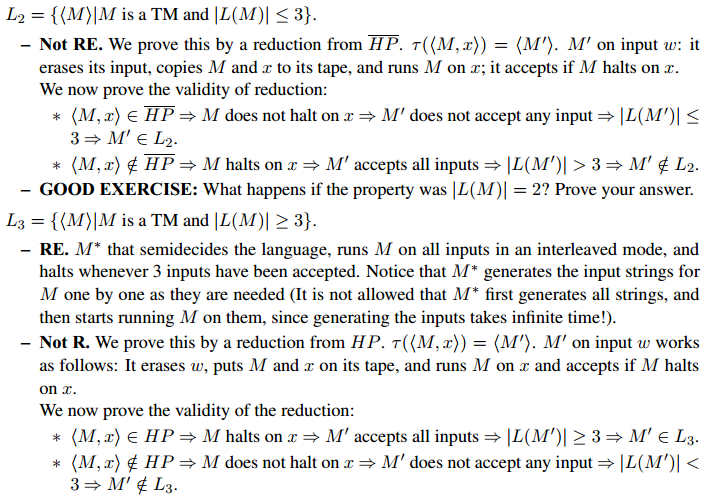countable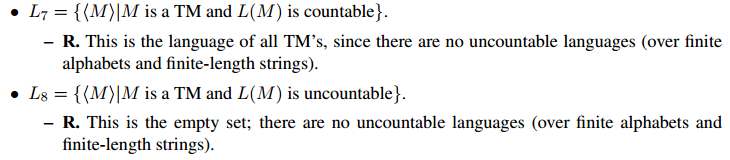RE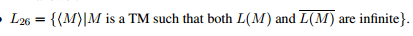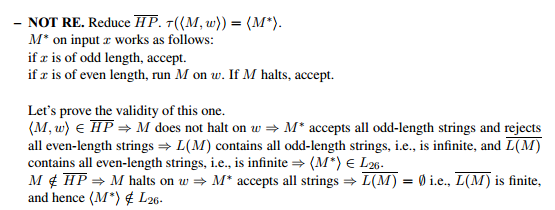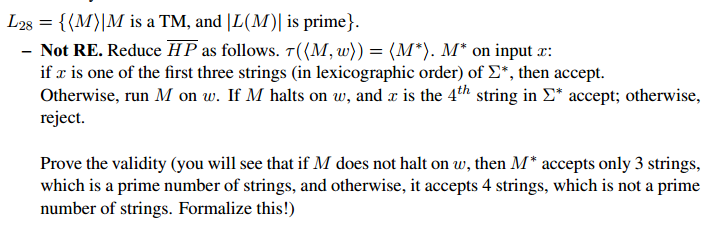展开全文• 计算理论导论中文版，美国麻省理工学院 Michael Sipser著。
• ## 图灵机与计算理论

千次阅读 2018-06-18 10:38:45
图灵机和计算理论是人工智能乃至整个计算机科学的理论基础，邱奇-图灵论题告诉我们一切可计算过程都可以用图灵机模拟。 图灵机 图灵机，又称图灵计算、图灵计算机，是由数学家艾伦·麦席森·图灵（1912～1954）...
前言

图灵机和计算理论是人工智能乃至整个计算机科学的理论基础，邱奇-图灵论题告诉我们一切可计算过程都可以用图灵机模拟。

图灵机

图灵机，又称图灵计算、图灵计算机，是由数学家艾伦·麦席森·图灵（1912～1954）提出的一种抽象计算模型，即将人们使用纸笔进行数学运算的过程进行抽象，由一个虚拟的机器替代人们进行数学运算。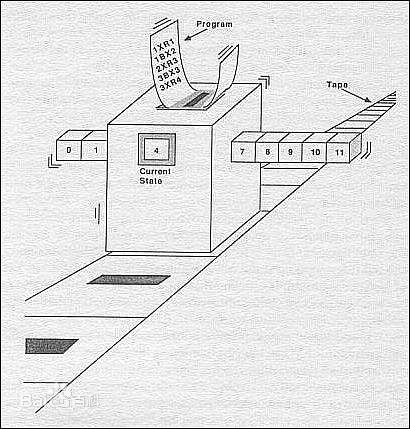图灵机指一个抽象的机器，它有一条无限长的纸带，纸带分成了一个一个的小方格，每个方格有不同的颜色。有一个机器头在纸带上移来移去。机器头有一组内部状态，还有一些固定的程序。在每个时刻，机器头都要从当前纸带上读入一个方格信息，然后结合自己的内部状态查找程序表，根据程序输出信息到纸带方格上，并转换自己的内部状态，然后进行移动。

每一个会决策、会思考的人都可以被抽象地看成一台图灵机。该模型主要有四要素：输入集合、输出集合、内部状态和固定的程序。如果把人进行抽象，那么输入集合就是所处环境中所看到、听到、闻到、感觉到的一切；输出集合就是人的每一言每一行，还有表情动作；内部状态集合则可以把神经细胞的状态组合看成一个内部状态，所有可能的状态集合将是天文数字。

人有记忆，图灵机有没有？有，它有了内部状态就可以看成有记忆，内部状态会记录所经历过的世界。

很多现象似乎都能被图灵机包括，如人了IDE情绪和情感，可以看成某种内部状态，心情好的情绪下，输入和输出是一套规则，而心情不好的情况下，输入输出又是另外一种规则。

无论是神经元传递信息、变化状态的规律都是固定的，可以被程序化。那么头脑作为神经元的整体，它的运作也比如遵循固定的规则，即程序。正如图灵相信的，人脑也不会超越图灵机模型。

关于图灵机的学习问题，看似图灵机不包括学习，因为学习就意味着程序的改变，而图灵机不能在运行过程中改变自己的程序。很有可能一个图灵机的规则没有改变，只不过激活了它的某些内部状态，因为发生了本质的变化，尽管给它相同的输入，它却有完全不同的输出，这样看起来似乎它会学习了，虽然图灵机的程序一点都没变。

通用图灵机存在吗

是否存在一台万能图灵机能模拟其他所有图灵机？这台万能图灵机就是通用图灵机，把输入x和图灵机m信息都一起输入到通用图灵机，就能计算出一个结果o，这样便可以模拟任何一台图灵机。

图灵机m信息其实就是编码，通过编码可以将事物进行编号，将所有图灵机进行编码后每个图灵机就有了描述信息，如果某台图灵机的编码为m，输入为x，则将两者合并一起输入到万能图灵机中，计算得到结果。

停机问题

尽管图灵机很强大，但它也有解决不了的问题，比如停机问题。通俗地讲，停机问题就是判断任意一个程序是否能在有限的时间之内结束运行的问题。该问题等价于如下的判定问题：是否存在一个程序P，对于任意输入的程序w，能够判断w会在有限时间内结束或者死循环。

1936年图灵已经证明了这样的程序是不存在的。

从停机问题中可以看到的确存在人类能构造出来而图灵机解决不了的问题，也就是计算机不能解决的问题，这类问题是不可计算的。停机问题揭示了宇宙中的某种共性，所有计算机解决不了的问题本质上讲都和图灵停机问题是计算等价的。相似问题还包括是否存在一个程序能检测所有程序会不会出错。

停机问题也喝复杂系统的不可预测性有关，存不存在一个程序接收某个复杂系统的规则，然后输出结果？答案是不可能。所以得到一个结论是我们要想弄清楚某个复杂系统运行结果，唯一的办法就是人为去编程实际运行后才能得到结果。这也说明了某些事机器做不了而人类能实现。

超越图灵计算理论

一个固定的程序不可能超越图灵计算理论的限制，但如果一个程序能在每时每刻都变化使之不再是原来的自己，那么就可以超越图灵计算理论。就好比人类每时每刻都经过细胞更新使得不再是原来的自己，所以人类超越图灵计算理论。这也就是说，人们没办法通过编写一个固定的程序来实现大脑功能。要实现真正的人工智能，就需要一个能不断改变自己的程序，而且这种改变也不是一个固定的程序。

————-推荐阅读————

我的2017文章汇总——机器学习篇

我的2017文章汇总——Java及中间件

我的2017文章汇总——深度学习篇

我的2017文章汇总——JDK源码篇

我的2017文章汇总——自然语言处理篇

我的2017文章汇总——Java并发篇

跟我交流，向我提问：公众号的菜单已分为“读书总结”、“分布式”、“机器学习”、“深度学习”、“NLP”、“Java深度”、“Java并发核心”、“JDK源码”、“Tomcat内核”等，可能有一款适合你的胃口。

为什么写《Tomcat内核设计剖析》

欢迎关注：展开全文人工智能 AI 图灵机 停机问题
• 昨天参加《计算理论导论》的学习，原本以为是第一次上课的，没想到几十天前，已经开始上了一天的课。一开始没仔细看清楚科目名，以为是计算机理论的，心想着不是很简单吗，就把一体计算机拆开来，一个个研究分析，...
• ## 计算理论导引答案

热门讨论 2008-06-11 10:02:13
本他以独特的视角，系统地介绍了计算理论的三个主要内容：自动机与语言、可计算性理论和计算复杂性理论。绝大部分内容是基本的，同时对可计算性和计算复杂性理论中的某些高级内容进行了重点介绍。内容很好，本书十分...
• ## 计算理论导引 答案

热门讨论 2008-01-02 23:43:08
本书由计算理论领域的知名权威Michael Sipser所撰写。他以独特的视角，系统地介绍了计算理论的三个主要内容：自动机与语言、可计算性理论和计算复杂性理论。绝大部分内容是基本的，同时对可计算性和计算复杂性理论中...
• 《计算机视觉 ——计算理论与算法基础》是马颂德、张正友编著的经典教材。本资源为高清完整版，分享给大家一同学习！
• 湖南大学研究生计算理论导引课件,参考书目是经典的计算理论导引那本书
• Marr的视觉计算理论立足于计算机科学，系统地概括了心理物理学、神经生理学、临床神经病理学等方面已取得的所有重要成果，是迄今为止最为系统的视觉理论。Marr 的视觉计算理论虽然在细节甚至在主导思想方面尚存在...
• 关于"计算"的核心讨论，并分析CPI引出指令集概念图灵机 冯诺依曼结构
• ## 计算理论重点——Theory of Computation

万次阅读 多人点赞 2013-01-11 15:54:57
一个学期的计算理论课程已经结束，给我的感觉吧，计算理论是一门计算机不得不学，学了短期又没用，但是可以培养一些逻辑思维的课程。其最关注的问题是什么是可计算性，什么问题可计算，问题之间的映射/归约，计算...
• 一本权威的教材，绝对正版，带目录，详细介绍了计算理论，是一本绝对实际好用的教材
• 计算理论 (3 weeks) 复杂理论(8 weeks) 先修内容： 离散数学，算法基础 What I can learn: 模型检测，计算理论数学基础，密码学应用 axiomatic system 公理化系统，公理化方法和因果化方法是西方世界最重要的...
• 一：视觉计算理论与算法研究（ 由×××自动化研究所马颂德等完成）"视觉计算理论与算法研究"的目标主要是研究计算机视觉，以使计算机具有通过二维图像感知三维环境信息的能力，包括感知、描述、理解和识别。...
• 转载自：计算理论导论第1章答案 想要完整答案文件建议到 原出处“转载自” 下载 转载自：计算理论导论第1章答案 想要完整答案文件建议到 原出处“转载自” 下载 ...
• ## 什么是可计算理论

千次阅读 2013-06-28 14:44:01
计算理论计算理论的一个分支，还有两个分支分别是自动机和计算复杂性。 这些名词都顾名思义，自动机讲的是计算的模型，比如图灵机，它是由无限长磁带，读写磁头和一些状态转移序列组成；可计算理论，研究的...
• 计算机是现代一种服务于人类的用于高度计算的机器 计算机由什么组成？ 硬件：硬盘 显示器 键盘 显卡。。。 看得见摸的着 软件：浏览器 QQ LOL wegame。。。。 看不见摸不着–》网站，服务器存在 2.计算机语言 ...windows
•ibm
• 本学期老师给我们上了一门研究生要上的课程：计算理论导引。 起初还不是很理解的，后来觉得从0到1的创造出一个具有思维的及其，这的确是无比的伟大。Turing机： 构造分为三部分： 1。一条带，一个读写头和一个...
• 计算理论基础PDF电子书 第二版 张立昂译 英文版2-5章部分答案（图片格式）
• 计算理论导引》是计算理论领域的经典著作，被国外多所大学选用用为教材。系统地介绍计算机理论的三大主要内容：自动机与语言、可计算性理论和计算复杂性理论。本书课后习题答案价值巨大，对于理解计算理论具有重要...
• 中文版的计算理论导引的课后答案，有些题目不是很全，可以去找英文的看看
• 计算理论导引第二版的课后习题答案，英文版的，但是很全...